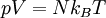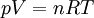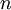# Difference between revisions of "Equation of State: Ideal Gas"

The ideal gas equation of state, developed by Benoît Paul Émile Clapeyron in 1834 (Ref. 1) is given by:$\left. p V = N k_B T \right.$

or$\left. p V = n R T \right.$

where:

•$p$ is the pressure
•$V$ is the volume
•$N$ is the number of molecules
•$n$ is the number of moles
•$T$ is the absolute temperature
•$k_B$ is the Boltzmann constant
•$R$ is the molar gas constant.